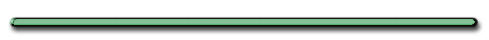HomeConservation of Momentum

Newton Collider

B) Recoil Problem - When interacting objects start from rest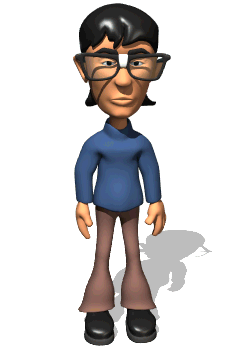"What is the total momentum before cannon is fired?" "Total momentum after clown is fired?"

[ Flash ]"Total momentum before = 0. (at rest) "Total momentum after  = 0. (momenta are equal but opposite.)""In real life, it would be foolish to shoot a rifle  like this.  Why?""The recoil on that rifle would throw the shooter's shoulder out!"

 Ex 1) A 4.0 kg rifle fires a 5.0 x 10-3 kg bullet at a velocity of 500. m/s.  What is the velocity acquired by the rifle?Ex 1) A 4.0 kg rifle fires a 5.0 x 10-3 kg bullet at a velocity of 500 m/s.  What is the velocity acquired by the rifle? (no shooter)

After rifle is fired

 Rifle Bullet mR = 4.0 kg Rifle mB =5.0 x 10-3 kg VR  = ? VB = 500. m/s

Total Momentum Before =
Total Momenta After Interaction

0 = m1V1 + m2V2

Subtract m2V2 from both sides

m1v1 = - m2V2

In problem Solving, remove
negative sign and use

 m1v1 = m2v2

(Not in Reference Table)

Ex 1) A 4.0 kg rifle fires a 5.0 x 10-3 kg bullet at a velocity of 500. m/sWhat is the velocity acquired by the rifle?

 Rifle Bullet mR = 4.0 kg mB =5.0 x 10-3 kg VR  = ? VB = 500. m/s

 m1v1 = m2v2

(4.0 kg)V =(5.0x10-3 kg)500.m/s

(4.0 kg)V = 2.5 kgm/s

V  =  .63 m/s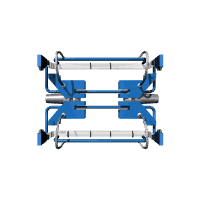Momenta before/after= 0 before

Equal Mass

W.F.U.

Total momentum after = 0 ??

Yes, the slower cart has a greater mass

Ex 2) Two disk magnets are arranged at rest on a frictionless horizontal surface.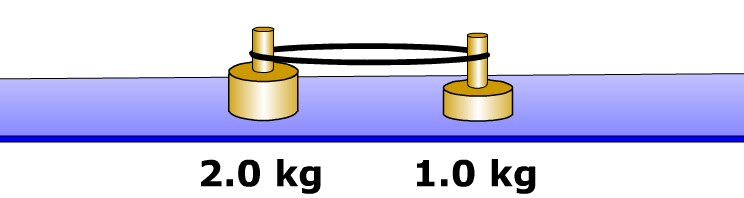When the string holding them together is cut, they move apart under a magnetic force of repulsion.

a) What is the total momentum of the magnets before the string is cut?Total momentum before = 0

(Both objects begin at rest)

b) What is the total momentum of the magnets after the string is cut?

Total momentum after = 0

(Total mom. before = Total mom. after)

c) What is the velocity of the 1.0 kg mass when the velocity of the 2.0 kg magnet is 30. m/s?

| Flash  |

m1 = 2.0 kg

m2 = 1.0 kg

V= ?

V1 = 30. m/s

m1v1 = m2v2

2.0 kg(30. m/s) = 1.0 kg(V)

V = 60. m/sWhat is the velocity of the cannon below?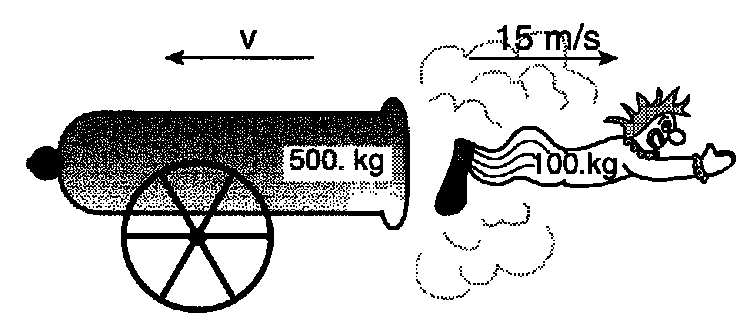m1v1 = m2v2

500 kg(V1) = 100 kg(15 m/s)

V1 = 3 m/sEnergyEnrichmentRifle Recoil
Dr. Dave's Hall of Fame